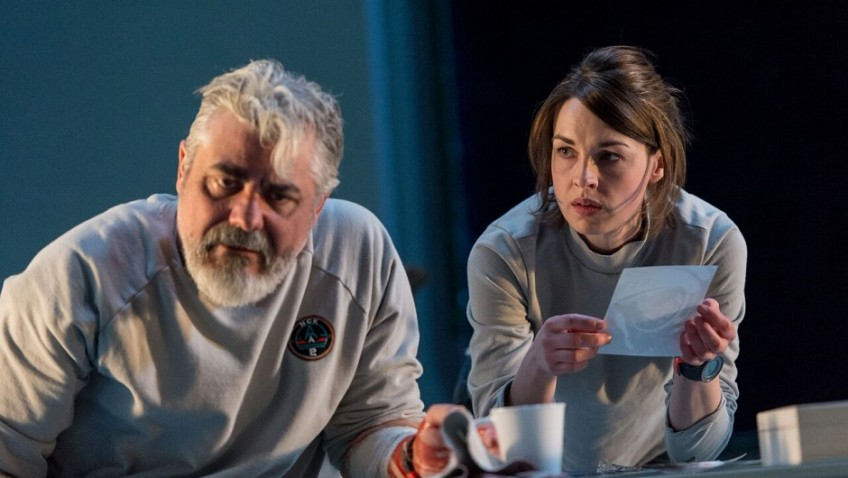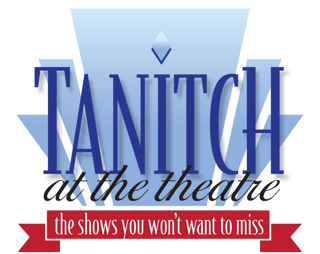# “X” may appeal to Sci-Fi fans but the cinema does this sort of thing so much better.

Robert Tanitch reviews X at Royal Court Theatre, London SW1

A British crew at a research station in space is orbiting round Pluto. They are unable to leave or send for help. They have lost contact with Earth

They are stranded billions of miles away, waiting for somebody (who will never come) to pick them up. They no longer know the time or the day.  There is nothing for them to do but lose their memories, lose their language and go mad and die.

Alistair McDowall’s X may appeal to Sci-If fans but the cinema does this sort of thing so much better.

The actors, headed by Jessica Raine, Darrell D’Silva and James Harkness, do a lot of shouting in Vicky Featherstone’s production and the second act goes completely to pieces.

Alistair McDowall is the author of the wildly over-praised Pomona. I think you can get some idea of what his new play X is like if I just quote very briefly from his longest speech.

This is only a very small part of what the actor actually has to say. The X’s run to nearly four pages of closely printed text:

X X X X X X X X X X X X X X X X X X X X X X X X X X X X X X X X X X X X X X X

X X X X X X X X X X X X X X X X X X X X X X X X X X X X X X X X X X X X X X X

X X X X X X X X X X X X X X X X X X X X X X X X X X X X X X X X X X X X X X X

X X X X X X X X X X X X X X X X X X X X X X X X X X X X X X X X X X X X X X XX X X X X X X X X X X X X X X X X X X X X X X X X X X X X X X X X X X X X X X

X X X X X X X X X X X X X X X X X X X X X X X X X X X X X X X X X X X X X X X

X X X X X X X X X X X X X X X X X X X X X X X X X X X X X X X X X X X X X X X